All New-Tons Of Fun Lab Stations: Day 2

15 teachers like this lesson
Print Lesson

Objective

Students will be able to observe a propulsion demonstration to determine which of Newton's Laws it represents.

Big Idea

Want to get your students excited about literacy? This lesson provides an exciting context to work on scientific reading, writing, speaking, and designing. Students complete fun labs and discussions with "launching" tennis balls and ruler flip races!

Introduction and Connection to the NGSS and Common Core

This is the second lesson of a unit focusing on scientific and engineering literacy. It builds off of protocols that students learned in the previous lesson. In this lesson, students apply discussion protocols and work on writing a scientific explanation. It may take two class periods to complete this lesson in its entirety, including the writing of the scientific paragraph and closure.

This lesson is designed to address the following NGSS and Common Core Standards:

MS-PS2-1      Apply Newton’s Third Law to design a solution to a problem involving the motion of two colliding objects.

MS-PS2-2      Plan an investigation to provide evidence that the change in an object’s motion depends on the sum of the forces on the object and the mass of the object

CCSS.ELA-LITERACY.RST.6-8.1  Cite specific textual evidence to support analysis of science and technical texts.

CCSS.ELA-LITERACY.WHST.6-8.1.B  Support claim(s) with logical reasoning and relevant, accurate data and evidence that demonstrate an understanding of the topic or text, using credible sources.

Scientific and Engineering Practices:

1 Asking Questions and Defining Problems   Students at any grade level should be able to ask questions of each other about the texts they read, the features of the phenomena they observe, and the conclusions they draw from their models or scientific investigations.

2 Developing and Using Models     Students should be able to develop and/or use a model to predict and/or describe phenomena.

6 Constructing Explanations and Designing Solutions     Construct a scientific explanation based on valid and reliable evidence obtained from sources (including the students’ own experiments) and the assumption that theories and laws that describe the natural world operate today as they did in the past and will continue to do so in the future.

Engaging in Argument from Evidence    Construct, use, and/or present an oral and written argument supported by empirical evidence and scientific reasoning to support or refute an explanation or a model for a phenomenon or a solution to a problem.

Crosscutting Concepts:

Systems and System Models: A system is an organized group of related objects or components; models can be used for understanding and predicting the behavior of systems.

*While this lesson focuses on creating models, the discussion in this lesson ask students to connect to all of the CCC's.

Connecting to the Essential Question: What are you supposed to learn today?

5 minutes

I begin every class by asking the students "What are you going to learn today?". Students respond by referring to the Essential Question, "How can I demonstrate science and engineering literacy?". This EQ is included on their Unit Plan and on the front board.

This lesson continues from the Day 1 lesson and helps students to gather knowledge used in the end of the unit to design bottle rockets.  In the previous lesson, students used models to engage in group discussions. The discussion protocols taught in that lesson continue in this lesson.

*The success of this lesson hinges on experiences gained in previous lesson.

As the previous lesson helped students to practice discussion protocols, thinking beyond the text, and in connecting to the NGSS Crosscutting Concepts, I ask students to reevaluate their self assessments on the skills included in the unit plan. Students rank themselves on each of the skills included in the Unit Plan.  Students rank themselves on a scale of 1 to 4 (4 being mastery). Students will continue to update these scores over the course of the unit. I emphasize to them that it is ok not to be at a "4". Learning is about growth! We will use this starting point to track the growth in their learning.

Notice in the student work below, that the student updates his scores over the course of the unit as he grows in his level of mastery.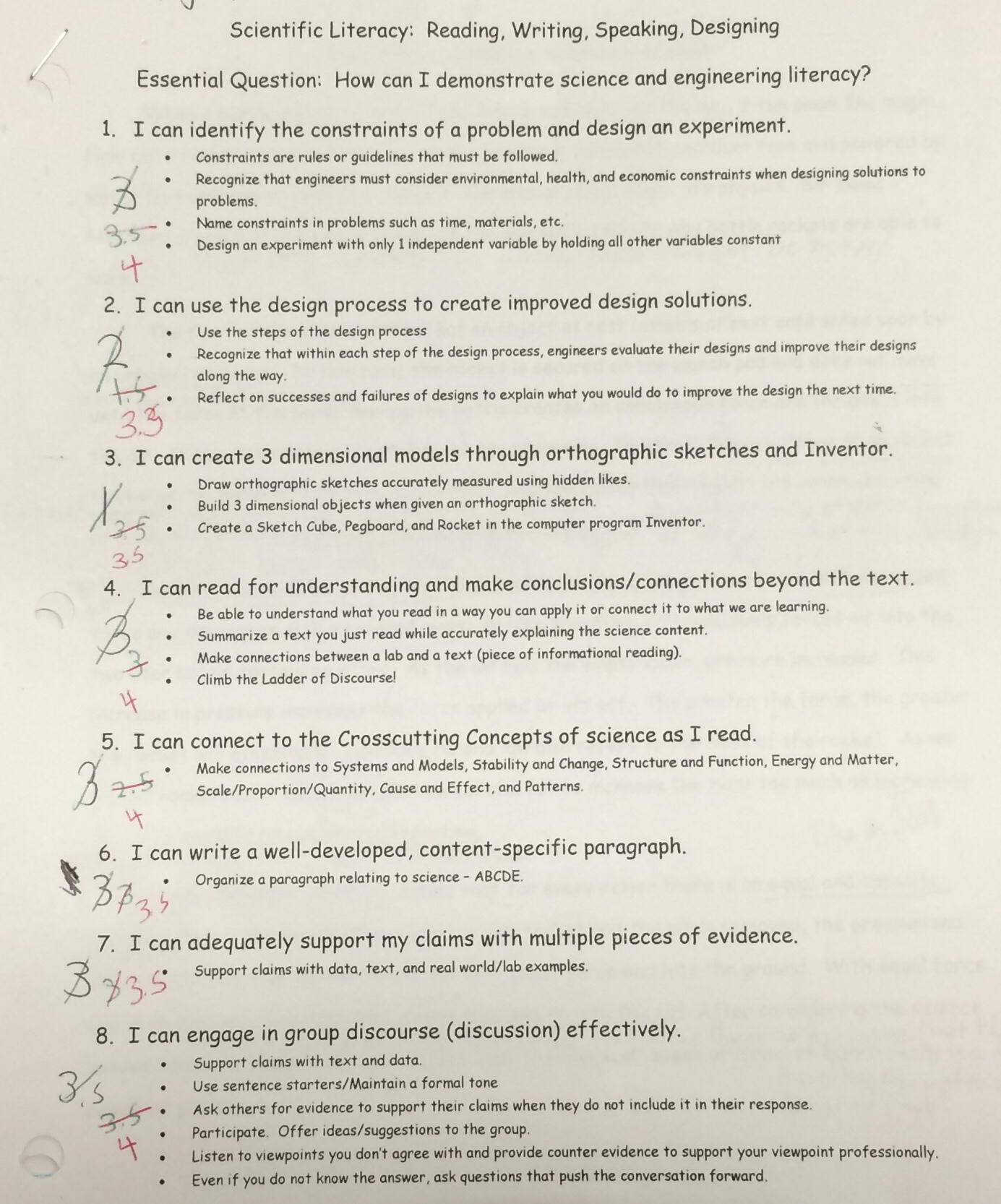Discussion Protocol #1 and Modeling: A Momentous Propulsion

25 minutes

Have students turn to their All New Tons of Fun Student Document (Get it?  All New-Tons (Newtons) of Fun Labs?) and explain that they will be going through some demonstrations that model Newton's Laws. Following each demonstration, students use the discussion protocols that we practiced yesterday. (It is important to note that students have already read a text focused on Newton's Laws and have practiced the protocols used in this section in Day 1's lesson. I have included the "Newton's Laws and Rocket Reading" in the resources.)

Have students complete the "A Momentous Propulsion" procedure:

1.  Put on a pair of goggles.

2.  Hold a tennis ball directly ontop a basketball at arms length.

3.  Drop the balls at the same time so that they drop on top of each other.

4.  Watch what happens to both of the balls!

5.  Repeat steps #1 – 4 while holding the basketball over the tennis ball.

Discussion Protocol:

Follow these steps when answering the discussion questions.  After each step, any student can add to or reflect on the comments that were made.

1. Student cites text to explain the law.  (“The text states that….”)
2. Student summarizes what was cited in the text. (“In other words, Newton’s ___ law means….”)
3. Student discusses how the law connects to this specific model using the vocabulary from the law. (“This model connects to Newton’s ____ law because….”)
4. Student explains how this concept can be seen in a real world scenario. (“This is similar to……” or “I have seen this in the real world when……”)

Discussion Questions:  You will answer the same discussion questions for each demonstration.

1. How can this demonstration represent a model of  Newton’s 1st law?
2. How can this demonstration represent a model of Newton’s 2nd law?
3. How can this demonstration represent a model of Newton’s 3rd law?

Students follow the protocol for each of the three discussion questions.  Below is a video of my students following the protocol for Newton's 2nd law.

Discussion Protocol #2: A Momentous Propulsion and the Crosscutting Concepts

15 minutes

Students then complete the second of the protocols, the "Crosscutting Concept Discussion Protocol" in the same manner.

Crosscutting Concepts Discussion Protocol:

1. Choose 2 Crosscutting Concepts per model/demonstration.  Vary the concepts you choose so that all concepts are addressed at some point during the demonstrations.
2. Locate the first Crosscutting Concept in the text.
3. Assign a time keeper. Take 30 seconds of silent think time to find connections between the model and the current Crosscutting Concept (time keeper use device to time).  These could be connections, explanations or questions you generate based on the crosscutting concept and the demonstration.
4. One student shares connection/question.
5. Another student summarizes what the first student shared. (“What I hear you saying is……”.)  The student that shared confirms that their idea was summarized accurately.
6. A different student connects the idea to the text. (“This example connects to this concept because…(points to and reads from text)….”)
7. Other students share their connections with the group.
8. Group writes at least one connection/question that was shared on a sticky note and adds it to the appropriate Crosscutting Concept poster.

Below is a video of a group of students following the protocol.  These students are just beginning in mastering this process, it is not perfect. Here are some things to note that you might see with your students as well:

1. First, I started filming after the 30 second wait time.  Students may try to skip that time, but it is so important.  Make sure students use the wait time!

2. After the student summarizes, the student who is "being summarized" should confirm if the "summarizer" accurately described the connection. I found that students will just nod their head "yes" even if the "summarizer" did not paraphrase/summarize correctly. What students who are paraphrasing tend to do is simply say their own idea. Emphasize that the "summarizer" is not giving their own idea, they are restating what the other student shared. In addition, the students that are "being summarized" need practice at being able to say, "Actually, what I was saying was...." or "You've got part of what I was saying.  It was also important that I said....".

3. The last step is connection to the text. In this video, the student identifies the word "cause" and "effect" from the example. But, he doesn't actually cite words from the actual text that the example relates to. It is important that students actually connect to the words in the text. Otherwise, students make their own meaning of the CCC's that aren't necessarily scientific.  For example, students take "cause and effect" to be as simple as identifying a cause and effect when it is so much more. It is about identifying if causes are correlational or causational and that they can be used to make predictions about systems. Simply identifying a cause and effect does not meet the rigor of the CCC.

4.  It is important to emphasize to students that they must use different crosscutting concepts than they did in the previous lesson. This way, students will have to connect to all of the concepts by the end of this lab series. Without this requirement, students find a comfort zone and repeat their favorite crosscutting concept over and over. For example, if students did not have to choose a different CCC with each station, you would end up with a poster filled with "Cause and Effect" ideas but no "Stability and Change" connections.

Following the discussion protocol, students write their crosscutting connections on a sticky note and place it on the appropriate poster in my classroom:Students connect to more Crosscutting Concepts than I show below.  I just wanted to give you a few examples for some insight into the ideas students can generate.

Structure and Function:  The students note that the arrangement of the basketball and tennis ball is key to the function of the system.Scale, Proportion, and Quantity:  Students here generate a question with an independent and dependent variable. They ask if changing the quantity of the balls will affect the height the balls would bounce.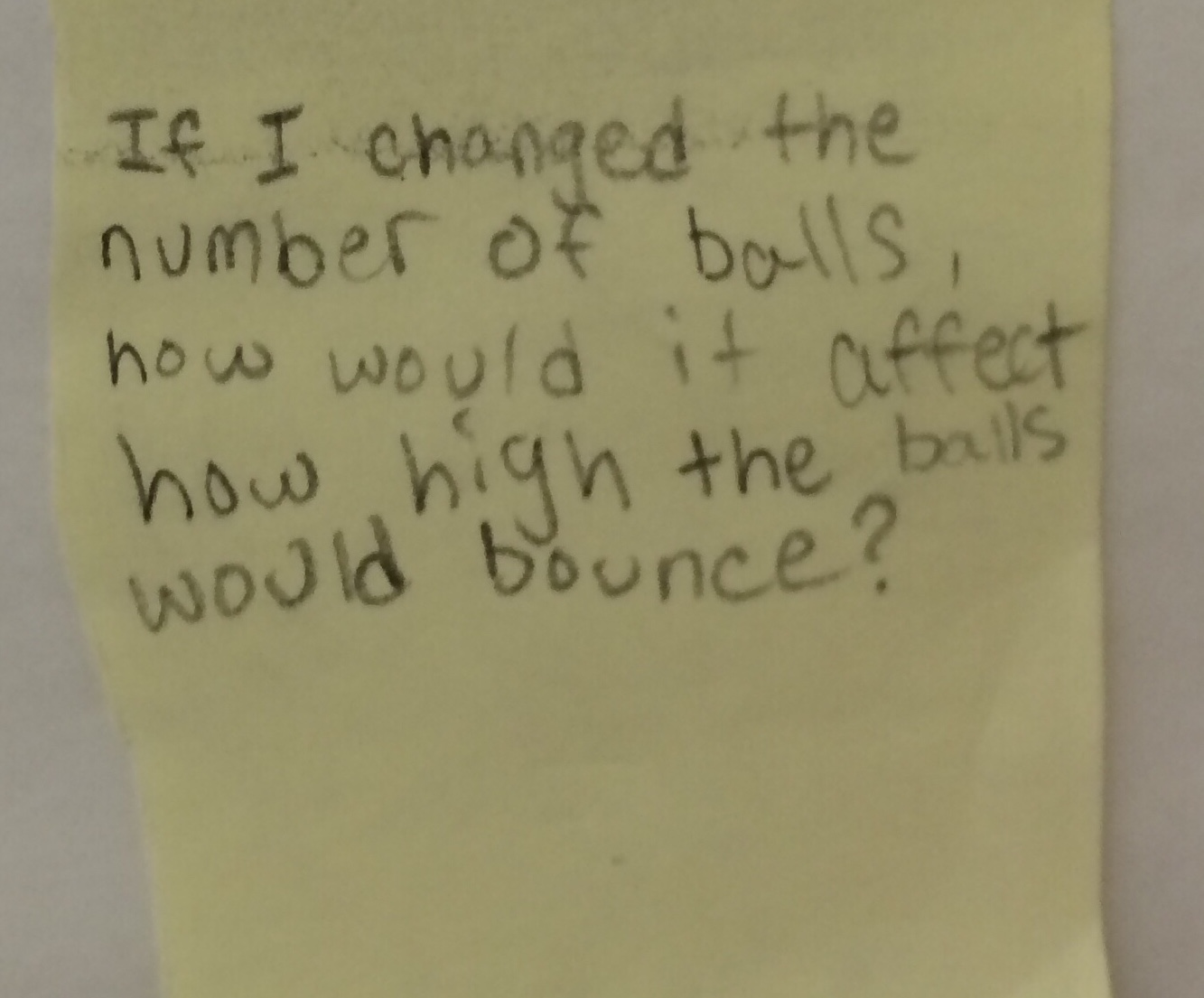Patterns:  Students noticed that when the basketball was on the bottom, the tennis ball flew higher than when the tennis ball was on the bottom. They used this pattern to make the general prediction that "when an object of more mass is on the bottom..."the object on top will go higher.".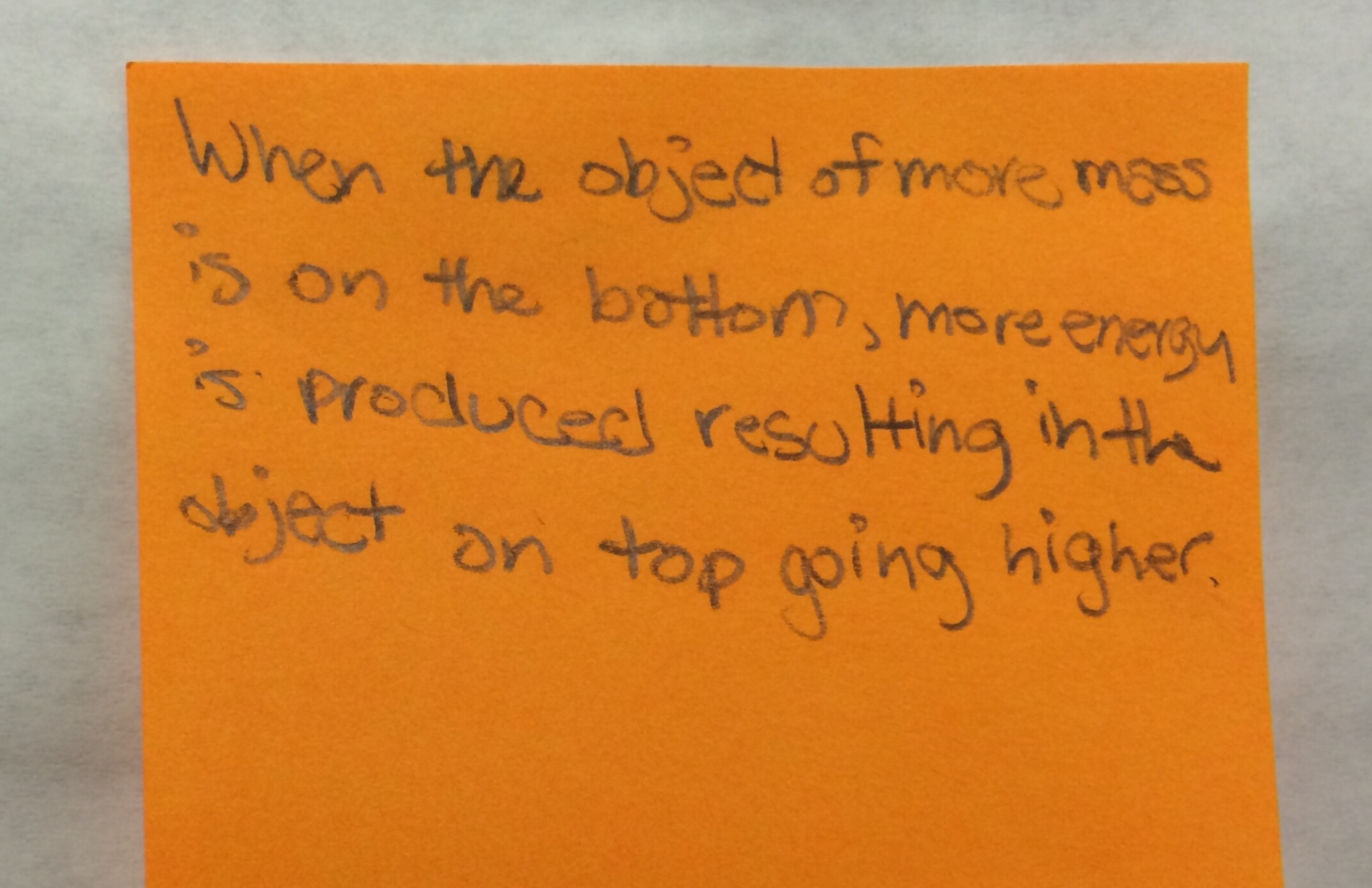Energy and Matter: Students here recognize that there are different types of energy that are involved in the reaction.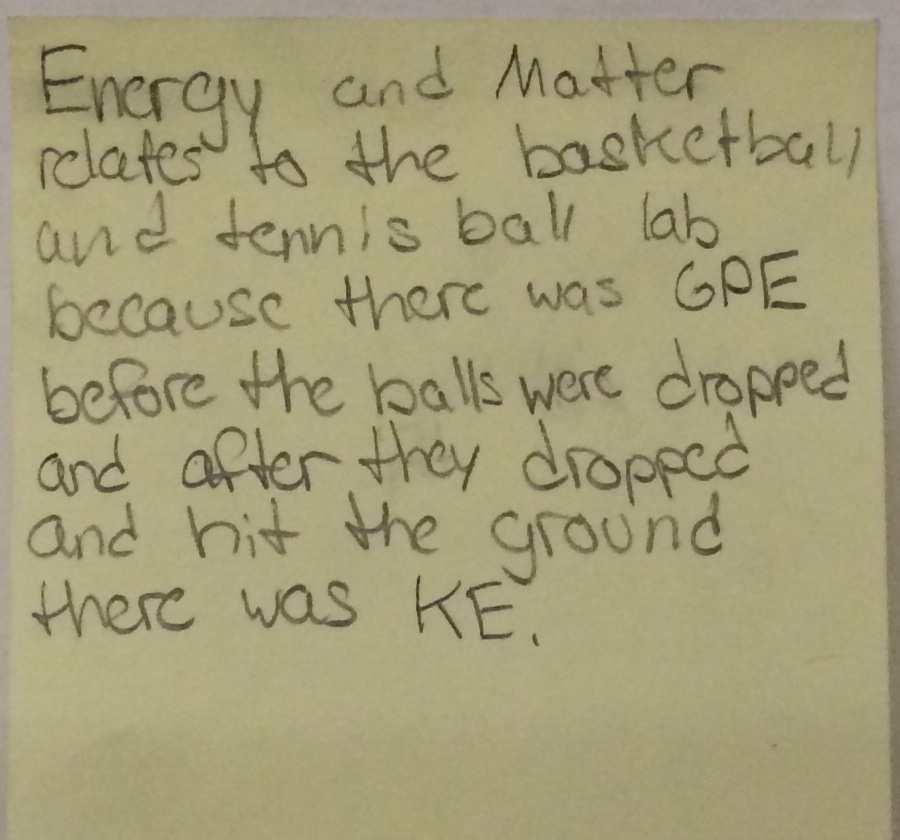Generating Questions and Using Mathematics: Marble Flip Races

25 minutes

After independently practicing the discussion protocols for Momentous Propulsion, explain that they will be completing the Marble Flip Lab. The protocol for this lab is different from the previous demonstrations. This lab focuses on generating a testable question/identifying constants and constructing a written explanation.

As I review the protocol with students, I stress that a key element is that they must generate a question that includes both an independent and dependent variable.  For example, students could not simply write, "How does mass affect it?". This type of sentence is missing a measurable dependent variable.  I explain that their Marble Flip question must be scientifically testable.  After generating the question, I tell them that they need to develop a list of constants needed in order to collect reliable data.

Students create their question and list it on a sticky note to place on the board labeled, "Generating Questions and Identifying Constraints/Constants". I have found that the key to students being able to design their own experiments is being able to construct a testable question and then isolating one variable. Having an awareness of all of the variables that must be held constant is the first step to being able to develop a procedure that can produce reliable data.Lab Protocol:

1. Read and follow the lab procedure.
2. Generate a question you could design an experiment about that includes both an independent and dependent variable.
3. Brainstorm as many variables as you can that will need to be held constant in order to produce reliable data if you were to design an experiment based on your question.
4. Write your question and the constants that you brainstormed on a large sticky note and add it to the designated board. (Be sure to add your names on the back of the sticky note.)
5. Answer written response question(s).

I ask, "If you were designing an experiment that would produce reliable data, why would it be important to consider the constants?" Students should respond with ideas such as, "You should only have one independent variable in the experiment.  If there is more, you won't know which variable will affect the results."

Students follow the procedure to complete the Marble Flip Lab:

1. Measure the masses of each of the three balls using a scale.  Record your measurements.

2. Set the ping pong ball at the starting line.

3. Line up the ruler with the starting line. Bend back the ruler to the tape mark on the floor. Flick the marble ball towards the finish line.

4. Once you have the method of “flicking” down, time the amount of time it takes for the ping pong ball to get to the finish line. Record your data.

5. Repeat steps 2 and 3 with a golf ball.

6. Repeat steps 2 and 3 with a tennis ball.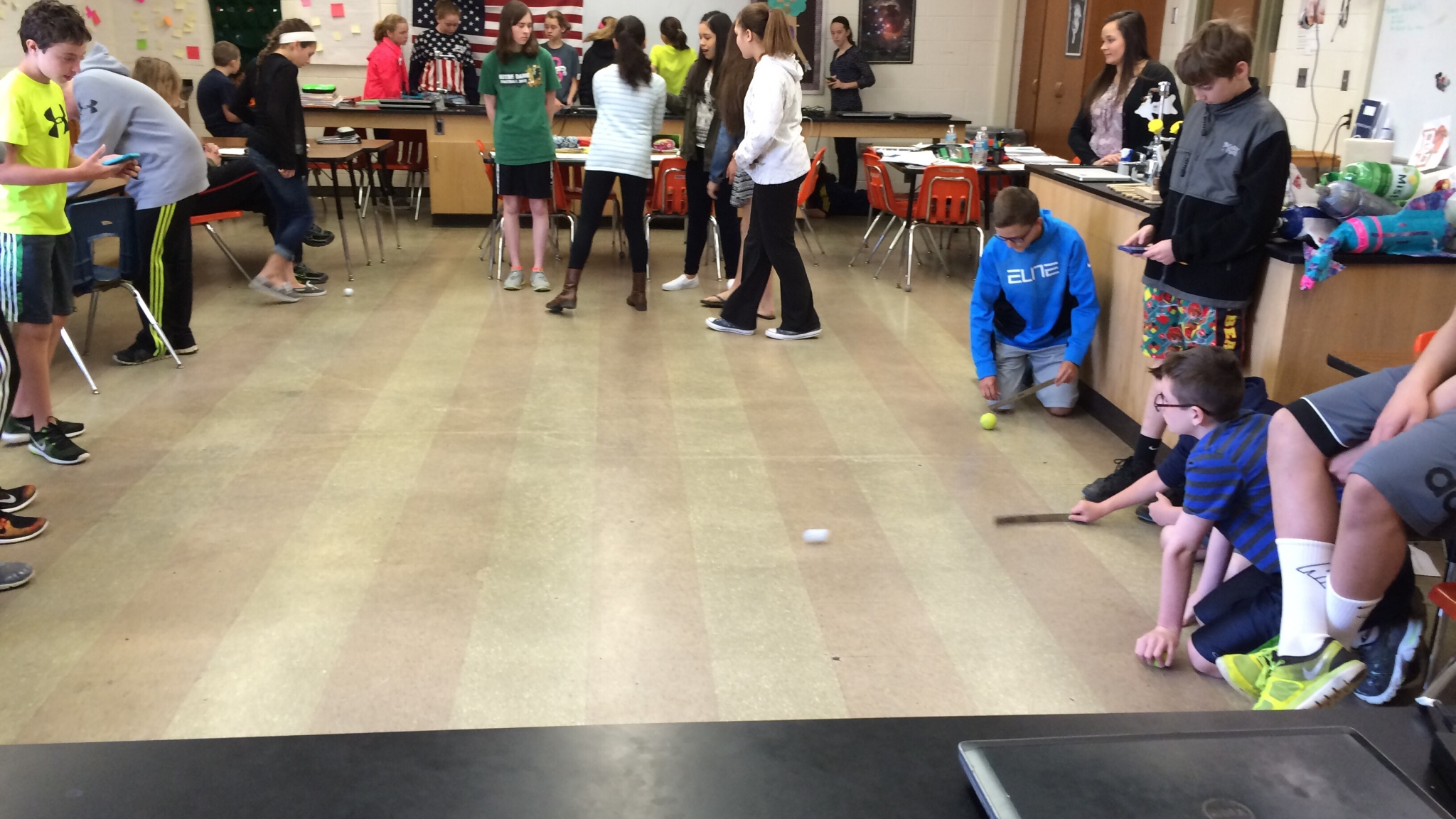Before completing the data table, students will need a review in how to substitute numbers into the velocity and acceleration formulas. Here are some important points I make to my students:

1. Velocity is distance divided by time. The data table says "3/time" because the distance we race is 3 meters.

2.  It is beneficial for students to see where the units for these measurements are derived. I explain that the unit for velocity is m/sec.  We look at the units for distance (meters) and the units for time (seconds). As the formula asks us to divide distance by speed, the units become m/sec.

3.  When looking at the acceleration formula, it is important to explain that "V initial" is the initial - starting - velocity and the "V final" is the final - ending - velocity.  I ask, "What is the velocity of the ball initially?" (0)

4.  A quick order of operations review follows (PEMDAS) so that students know that they must calculate the differences between the initial and final velocity first, before dividing by the seconds.

5.  Your math stars may be wondering, "Why is the unit for acceleration m/sec2? We don't square anything when doing the formula."  I explain that the unit for velocity is m/sec.  I write meters in the numerator of a fraction and seconds in the denominator of a fraction. I ask students to remember the procedure used when dividing fractions (multiply by the reciprocal.). "So what the acceleration formula is asking us to do is multiply m/seconds by 1/seconds.  Then, I multiply the fractions to show that "m x 1" is "m" and "seconds x seconds" is "seconds squared."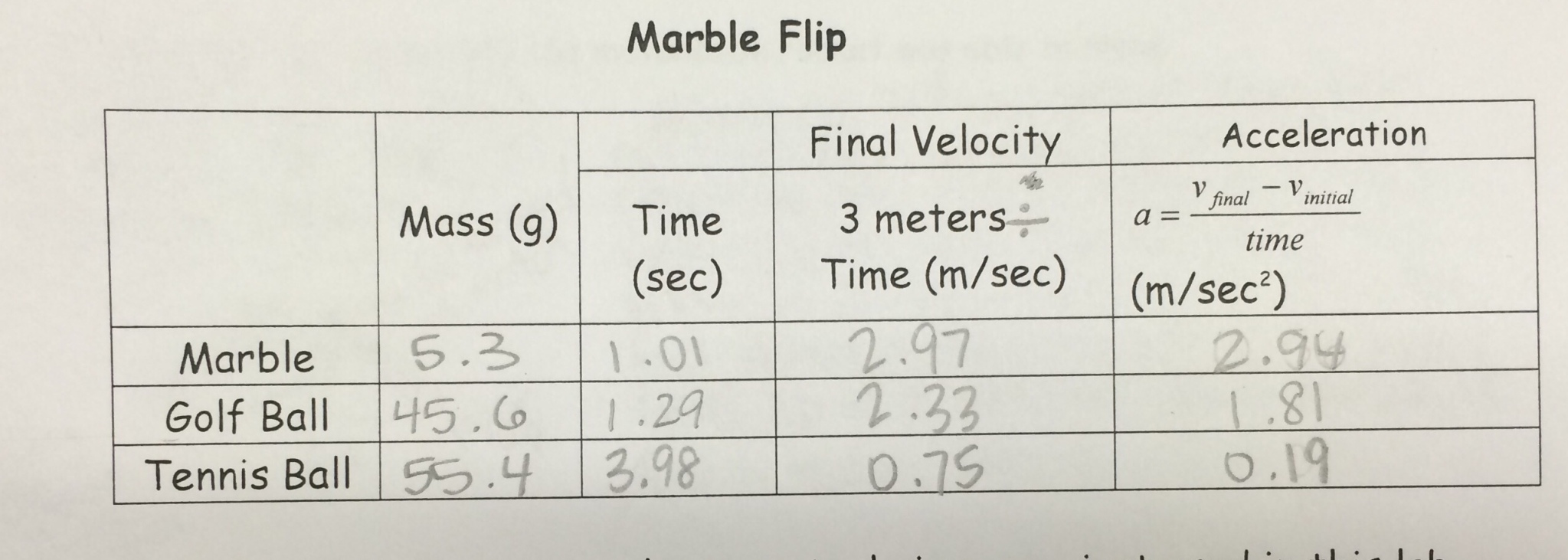After collecting data and calculating the results, students generate a testable question and brainstorm a list of variables they would have to keep constant to produce reliable data. Below, the student asks, "How does mass affect acceleration?". Students note that they should use the same ruler, the same pullback angle, the same surface, and same phone to time. These are constants that most students come up with. The student below shows a deeper understanding, recognizing that if they were going to only test for mass, they would also need to use balls of the same volume and made of the same materials. They recognize the flaw in the design of the original lab is that while the golf ball, tennis ball, and marble have differing masses, they also have different volumes and are made of different materials. Both of these variables could skew the data. Recognizing these factors is evidence of mastery.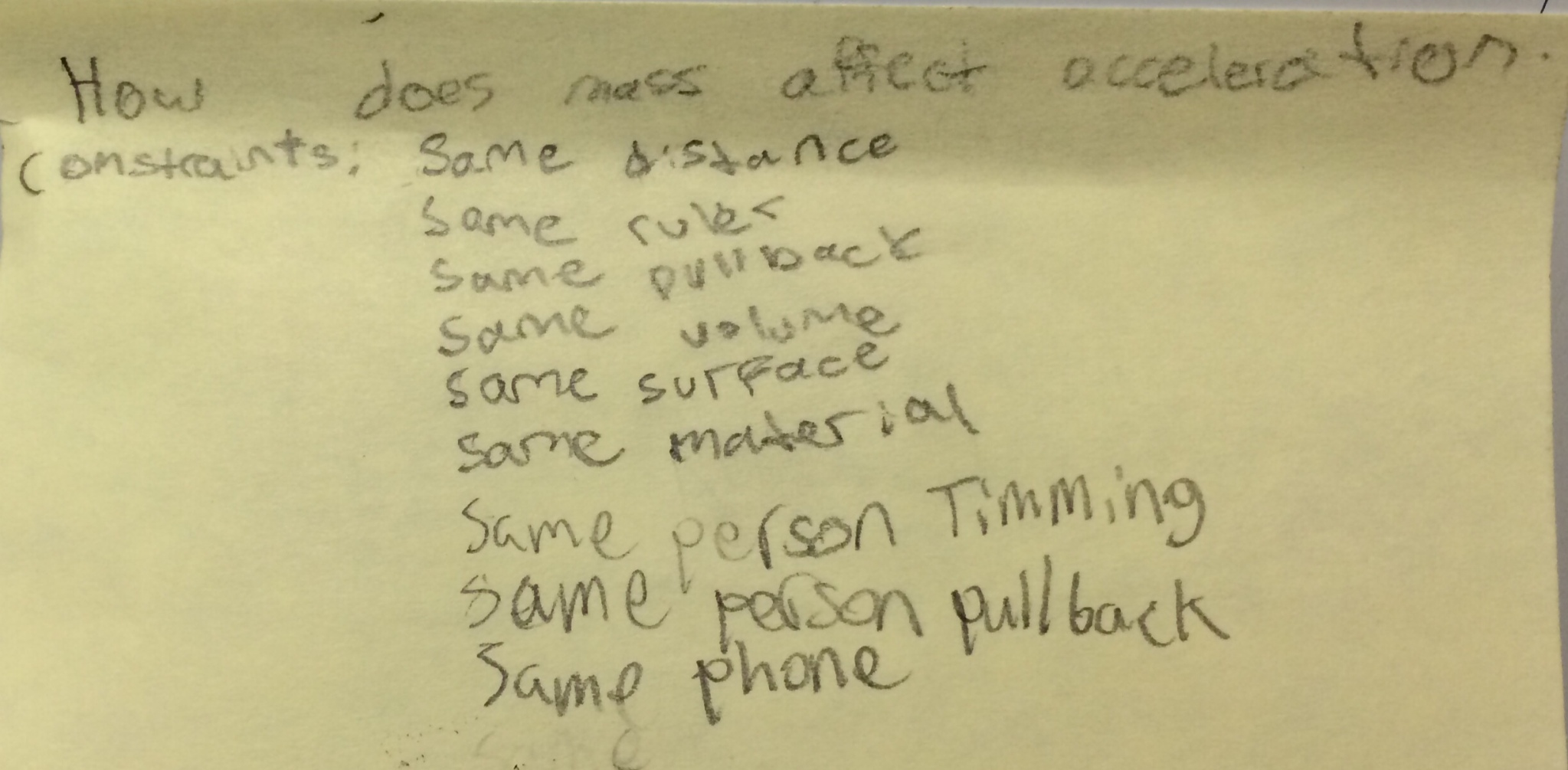The great thing about teaching students the Crosscutting Concepts is that students begin to apply them to labs even when they are not asked to do so. I walk by a group discussing the data they collected, finding them analyzing data as they applied the NGSS Crosscutting Concept of "Scale, Proportion, and Quantity". A student thinking about the data says, "Look at the jump in data. This data doesn't look proportional."

The video below is evidence of how the teaching science using the "three legged stool" of the Science and Engineering Practices, Crosscutting Concepts, and Disciplinary Core Ideas can foster real success in student learning!

Marble Flip: Constructing Written Explanations and Using Data as Evidence

20 minutes

After completing the Marble Flip Lab, students must construct a written explanation.  In my class, we use the format "ABCDE".  Below I include a description of each part as well as a connection to the student work shown below.

A - Assertion: Make a claim.  ("If Saline had a flip race, then the best choice would be the marble.")

B - Background: Provide the reader with background they will need to understand the arguments you are going to present.  ("In the lab, students hit various sized balls with a ruler and timed their time for a certain distance.)

C - Citation

a.  Cite text. (According to Newton's 2nd Law on page 2, it states that the acceleration of an object increases with increased force and decreases with increased mass.)

b.  Cite data.  More detailed information about what students need to do to demonstrate mastery when citing data is included in the "Closure" section.  ("According to "Marble Flip", the marble weighed 4.9 grams and the tennis ball weighed 57 grams.  The acceleration of the marble was 25.9 m/secand the acceleration of the tennis ball was .65 m/sec2.")

D - Discussion: Explain how your citations support your claim.  ("The marble had a faster acceleration because it had a decreased mass.")

E - End: Conclusion sentence. ("In conclusion, if Saline had a Flip Race, then the marble would be the be the best choice for the ball.")

For more detailed information about ABCDE paragraphs, check out the Quick Guide to the ABCDE Paragraph in the resource bin.Have students that struggle with organizing a paragraph even with the ABCDE format?  I have included an adapted version that I have created for students like this.  I use it as a scaffold.  Students use this "shell" until they grasp the pattern of the ABCDE paragraph, then they can write paragraphs on their own as they progress.  The purpose of this is to build their understanding of how to organize a paragraph, not to make this a "fill in the blank" sheet.  As students use the adapted version, meet/conference with them and help them connect to how the "shell" connects to the ABCDE paragraph.   After practice, students can remove this scaffold and write the paragraphs on their own!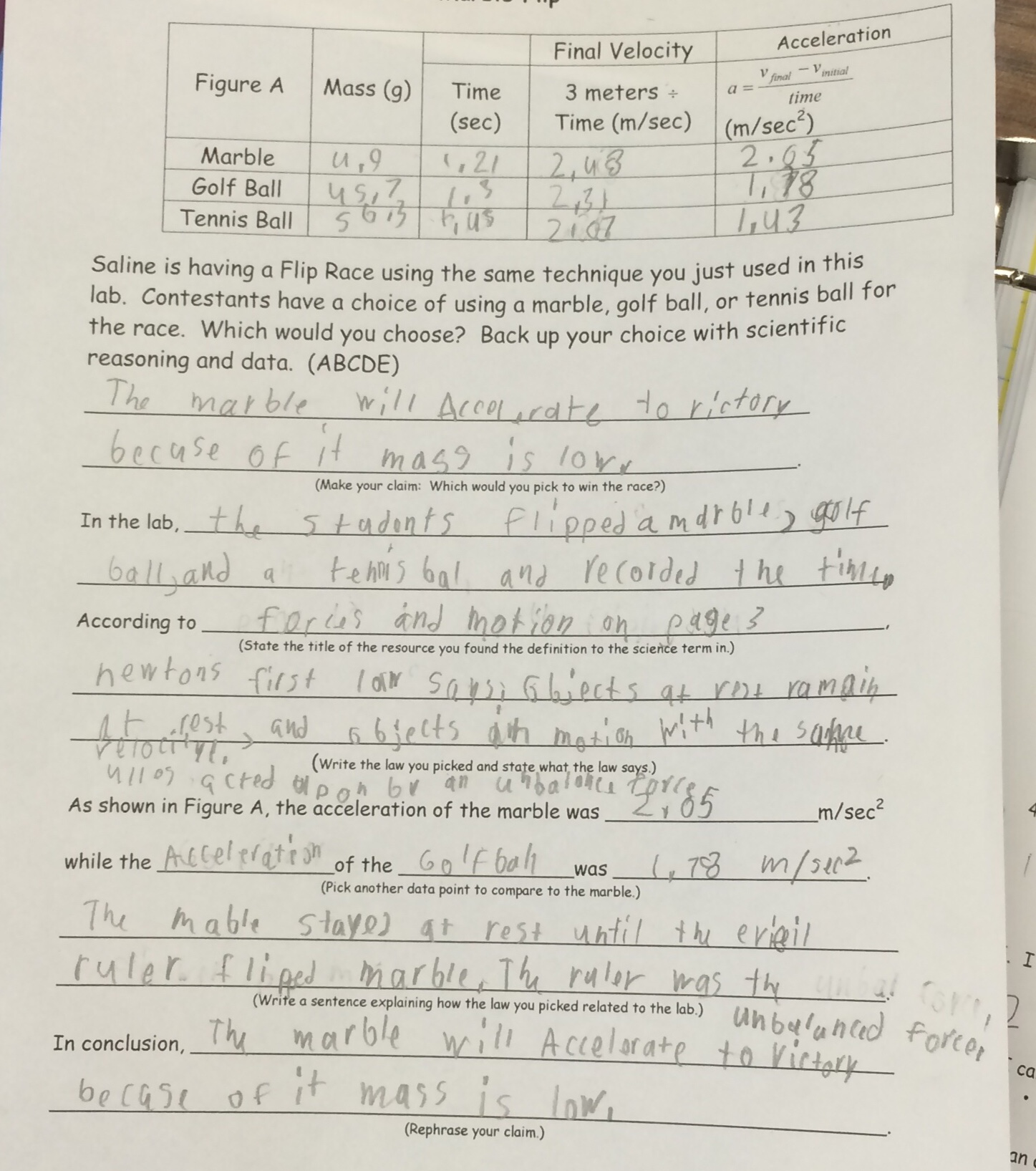Closure: How do you cite data as evidence?

15 minutes

Explain to the students that these are student responses to a question about the Marble Flip Lab they just completed.  Explain that these student responses are not in ABCDE format as their answers are.  I explain that we will be evaluating these students' work solely on how they used data as evidence.  I emphasize that we are not evaluating their handwriting, spelling, etc.  We are only focusing on their ability to use data effectively as evidence.

I provide each small group (2 - 3) with these responses on numbered slips of paper.  Then, I ask students to order them from "most effective use of data" to "weakest use of data".  Students discuss their reasoning and each group comes to a consensus on the order of the student work.  Then, I ask the students to generate criteria they found were important to effectively use data.

While students can easily "sort" student work from most effective to least effective, it is not natural for them to identify criteria.  Actually putting their finger on what makes writing effective is challenging.  However, it is critical.  Without this skill, they will not be able to adequately edit their own work.  Without this crucial step, I have found that students still cannot make improvements to their own work effectively.  In this video, the student perfectly ordered the student work; however, she is having trouble understanding exactly what it means to identify criteria.  After this conversation, she had an "ah-ha!" moment and generated an awesome criteria list!

I have included a Marble Flip Responses and Descriptors resource that goes through the strengths and weaknesses of each response.

After sorting, we generate a list as a class of the criteria needed.  I write these on the board and then I ask students to take a look at their own Marble Flip answers and see where they think their use of data would fall if it had been used as an example.  Last, students are provided with time to edit any aspect of their response that they feel does not meet the criteria.